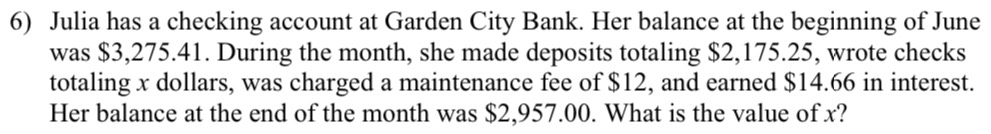### ¿Todavía tienes preguntas de matemáticas?

Pregunte a nuestros tutores expertos
Algebra
Pregunta6) Julia has a checking account at Garden City Bank. Her balance at the beginning of June was $$\ 3,275.41$$ . During the month, she made deposits totaling $$\ 2,175.25 ,$$ wrote checks totaling $$x$$ dollars, was charged a maintenance fee of $$\ 12 ,$$ and earned $$\ 14.66$$ in interest. Her balance at the end of the month was $$\ 2,957.00$$ . What is the value of $$x ?$$